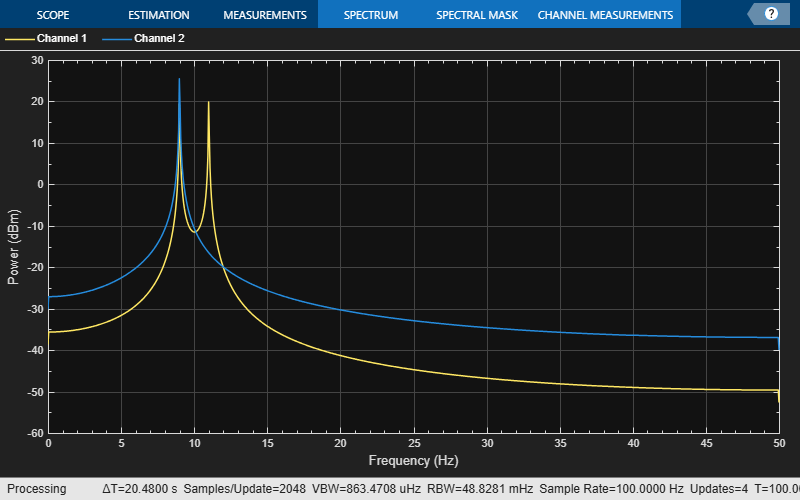Documentation

### This is machine translation

Mouseover text to see original. Click the button below to return to the English version of the page.

# ammod

Amplitude modulation

## Syntax

```y = ammod(x,Fc,Fs) y = ammod(x,Fc,Fs,ini_phase) y = ammod(x,Fc,Fs,ini_phase,carramp) ```

## Description

`y = ammod(x,Fc,Fs)` uses the message signal `x` to modulate a carrier signal with frequency `Fc` (Hz) using amplitude modulation. The carrier signal and `x` have sample frequency `Fs` (Hz). The modulated signal has zero initial phase and zero carrier amplitude, so the result is suppressed-carrier modulation.

### Note

The `x`, `Fc`, and `Fs` input arguments must satisfy `Fs` > 2(`Fc` + `BW`), where `BW` is the bandwidth of the modulating signal `x`.

`y = ammod(x,Fc,Fs,ini_phase)` specifies the initial phase in the modulated signal `y` in radians.

`y = ammod(x,Fc,Fs,ini_phase,carramp)` performs transmitted-carrier modulation instead of suppressed-carrier modulation. The carrier amplitude is `carramp`.

## Examples

collapse all

Set the sample rate to 100 Hz. Create a time vector 100 seconds long.

```fs = 100; t = (0:1/fs:100)'; ```

Set the carrier frequency to 10 Hz. Generate a sinusoidal signal.

```fc = 10; x = sin(2*pi*t); ```

Modulate `x` using single- and double-sideband AM.

```ydouble = ammod(x,fc,fs); ysingle = ssbmod(x,fc,fs); ```

Create a spectrum analyzer object to plot the spectra of the two signals. Plot the spectrum of the double-sideband signal.

```sa = dsp.SpectrumAnalyzer('SampleRate',fs, ... 'PlotAsTwoSidedSpectrum',false, ... 'YLimits',[-60 40]); step(sa,ydouble) ```Plot the single-sideband spectrum.

```step(sa,ysingle) ```